# Introduction

The R Bayesian evidence synthesis Tools (RBesT) have been created to facilitate the use of historical information in clinical trials. Once relevant historical information has been identified, RBesT supports the derivation of informative priors via the Meta-Analytic-Predictive (MAP) approach  and the evaluation of the trial’s operating characteristics. The MAP approach performs a standard meta-analysis followed by a prediction for the control group parameter of a future study while accounting for the uncertainty in the population mean (the standard result from a meta-analysis) and the between-trial heterogeneity. Therefore, RBesT can also be used as a meta-analysis tool if one simply neglects the prediction part.

Let’s consider a Novartis Phase II study in ankylosing spondylitis comparing the Novartis test treatment secukinumab with placebo . The primary efficacy endpoint was percentage of patients with a 20% response according to the Assessment of SpondyloArthritis international Society criteria for improvement (ASAS20) at week 6. For the control group, the following historical data were used to derive the MAP prior:

study n r
Study 1 107 23
Study 2 44 12
Study 3 51 19
Study 4 39 9
Study 5 139 39
Study 6 20 6
Study 7 78 9
Study 8 35 10

This dataset is part of RBesT and available after loading the package in the data frame AS.

RBesT supports all required steps to design a clinical trial with historical information using the MAP approach.

# Prior Derivation

## Meta-Analytic-Predictive Analysis

The gMAP function performs the meta-analysis and the prediction, which yields the MAP prior. The analysis is run using stochastic Markov-Chain-Monte-Carlo with Stan. In order to make results exactly reproducible, the set.seed function must be called prior to calling gMAP .

A key parameter in a meta-analysis is the between-trial heterogeneity parameter $$\tau$$ which controls the amount of borrowing from historical information for the estimation of the population mean will occur. As we often have only few historical trials, the prior is important. For binary endpoints with an expected response rate of 20%-80% we recommend a conservative HalfNormal(0,1) prior as a default. Please refer to the help-page of gMAP for more information.

The gMAP function returns an analysis object from which we can extract information using the functions from RBesT. We do recommend to look at the graphical model checks provided by RBesT as demonstrated below. The most important one is the forest plot, with solid lines for the MAP model predictions and dashed lines for the stratified estimates. For a standard forest plot without the shrinkage estimates please refer to the forest_plot function in RBesT.

library(RBesT)
set.seed(34563)
map_mcmc <- gMAP(cbind(r, n-r) ~ 1 | study,
data=AS,
tau.dist="HalfNormal",
tau.prior=1,
beta.prior=2,
family=binomial)
## Assuming default prior location   for beta: 0
print(map_mcmc)
## Generalized Meta Analytic Predictive Prior Analysis
##
## Call:  gMAP(formula = cbind(r, n - r) ~ 1 | study, family = binomial,
##     data = AS, tau.dist = "HalfNormal", tau.prior = 1, beta.prior = 2)
##
## Exchangeability tau strata: 1
## Prediction tau stratum    : 1
## Maximal Rhat              : 1
##
## Between-trial heterogeneity of tau prediction stratum
##   mean     sd   2.5%    50%  97.5%
## 0.3800 0.2050 0.0533 0.3500 0.8840
##
## MAP Prior MCMC sample
##  mean    sd  2.5%   50% 97.5%
## 0.258 0.086 0.113 0.249 0.465
## a graphical representation of model checks is available
pl <- plot(map_mcmc)

## a number of plots are immediately defined
names(pl)
##  "densityThetaStar"     "densityThetaStarLink" "forest_model"
## forest plot with model estimates
print(pl$forest_model)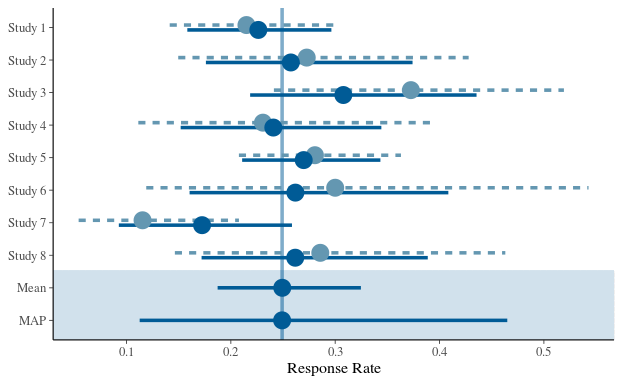An often raised concern with a Bayesian analysis is the choice of the prior. Hence sensitivity analyses may sometimes be necessary. They can be quickly performed with the update function. Suppose we want to evaluate a more optimistic scenario (with less between-trial heterogeneity), expressed by a HalfNormal(0,1/2) prior on $$\tau$$. Then we can rerun the original analysis, but with modified arguments of gMAP: set.seed(36546) map_mcmc_sens <- update(map_mcmc, tau.prior=1/2) ## Assuming default prior location for beta: 0 print(map_mcmc_sens) ## Generalized Meta Analytic Predictive Prior Analysis ## ## Call: gMAP(formula = cbind(r, n - r) ~ 1 | study, family = binomial, ## data = AS, tau.dist = "HalfNormal", tau.prior = 1/2, beta.prior = 2) ## ## Exchangeability tau strata: 1 ## Prediction tau stratum : 1 ## Maximal Rhat : 1 ## ## Between-trial heterogeneity of tau prediction stratum ## mean sd 2.5% 50% 97.5% ## 0.3330 0.1750 0.0331 0.3180 0.7230 ## ## MAP Prior MCMC sample ## mean sd 2.5% 50% 97.5% ## 0.2550 0.0769 0.1180 0.2500 0.4390 ## Parametric Approximation As a next step, the MAP prior, represented numerically using a large MCMC simulation sample, is converted to a parametric representation with the automixfit function. This function fits a parametric mixture representation using expectation-maximization (EM). The number of mixture components to best describe the MAP is chosen automatically. Again, the plot function produces a graphical diagnostic which allows the user to assess whether the marginal mixture density (shown in black) matches well with the histogram of the MAP MCMC sample. map <- automixfit(map_mcmc) print(map) ## EM for Beta Mixture Model ## Log-Likelihood = 4451.035 ## ## Univariate beta mixture ## Mixture Components: ## comp1 comp2 ## w 0.6591782 0.3408218 ## a 16.5925203 3.1555842 ## b 49.8328476 8.3667384 plot(map)$mix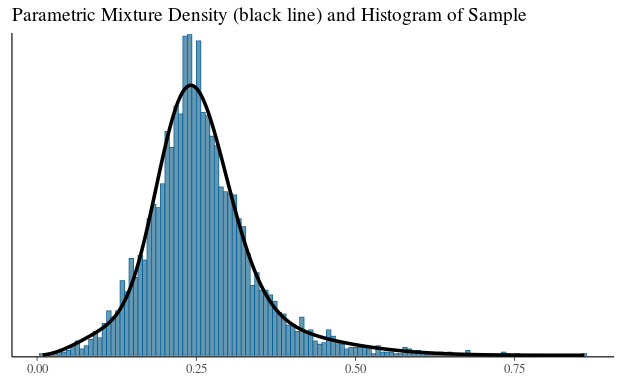## Effective Sample Size

The (usual) intended use of a (MAP) prior is to reduce the number of control patients in the trial. The prior can be considered equivalent to a number of experimental observations, which is called the effective sample size (ESS) of the prior. It can be calculated in RBesT with the ess function. It should be noted, however, that the concept of ESS is somewhat elusive. In particular, the definition of the ESS is not unique and multiple methods have therefore been implemented in RBesT. The default method in RBesT is the elir approach  which results in reasonable ESS estimates. The moment matching approach leads to conservative (small) ESS estimates while the Morita  method tends to estimates liberal (large) ESS estimates when used with mixtures:

round(ess(map, method="elir"))   ## default method
##  35
round(ess(map, method="moment"))
##  25
round(ess(map, method="morita"))
##  56

The Morita approach uses the curvature of the prior at the mode and has been found to be sensitive to a large number of mixture components. From experience, a realistic ESS estimate can be obtained with the elir method which is the only method which is predictively consistent, see  for details.

## Robustification of the MAP Prior

Finally, we recommend to robustify  the prior which protects against type-I error inflation in presence of prior-data conflict, i.e. if the future trial data strongly deviate from the historical control information.

## add a 20% non-informative mixture component
map_robust <- robustify(map, weight=0.2, mean=1/2)
print(map_robust)
## Univariate beta mixture
## Mixture Components:
##   comp1      comp2      robust
## w  0.5273425  0.2726575  0.2000000
## a 16.5925203  3.1555842  1.0000000
## b 49.8328476  8.3667384  1.0000000

Note that robustification does reduce the ESS.

round(ess(map_robust))
##  24

# Design Evaluation

Now we have a prior which can be specified in the protocol. The advantage of using historical information is the possible reduction of the placebo patient group. The sample size of the control group is supplemented by the historical information. The reduction in placebo patients can be about as large as the ESS of the MAP prior.

In the following, we compare designs with different sample sizes and priors for the control group. The comparisons are carried out by evaluating standard Frequentist operating characteristics (type-I error, power). The scenarios are not exhaustive, but rather specific ones to demonstrate the use of RBesT for design evaluation.

## Operating Characteristics

We consider the 2-arm design of the actual Novartis trial in ankylosing spondylitis . This trial tested 6 patients on placebo against 24 patients on experimental treatment. Success was declared whenever the condition

$\Pr(p_{treat} - p_{placebo} > 0) > 0.95$

was met. A MAP prior was used for the placebo response rate parameter. Here we evaluate a few design options as an example.

The operating characteristics are setup in RBesT in a stepwise manner:

1. Definition of priors for each arm.
2. Definition of the decision criterion using the decision2S function.
3. Specification of design options with the oc2S function. This includes the overall decision function and per arm the prior and the sample size to use.
4. The object from step 3 is then used to calculate the operating characteristics.

Note that for a 1-sample situation the respective decision1S and oc1S function are used instead.

### Type I Error

The type I can be increased compared to the nominal $$\alpha$$ level in case of a conflict between the trial data and the prior. Note, that in this example the MAP prior has a 95% interval of about 0.1 to 0.5.

p_truth       <- seq(0.1,0.95,by=0.01)
uniform_prior <- mixbeta(c(1,1,1))
treat_prior   <- mixbeta(c(1,0.5,1)) # prior for treatment used in trial
lancet_prior  <- mixbeta(c(1,11,32)) # prior for control   used in trial
decision      <- decision2S(0.95, 0, lower.tail=FALSE)

design_uniform   <- oc2S(uniform_prior, uniform_prior, 24, 6, decision)
design_nonrobust <- oc2S(treat_prior,   map          , 24, 6, decision)
design_robust    <- oc2S(treat_prior,   map_robust   , 24, 6, decision)

typeI_uniform   <- design_uniform(  p_truth, p_truth)
typeI_nonrobust <- design_nonrobust(p_truth, p_truth)
typeI_robust    <- design_robust(   p_truth, p_truth)

ocI <- rbind(data.frame(p_truth=p_truth, typeI=typeI_robust,    prior="robust"),
data.frame(p_truth=p_truth, typeI=typeI_nonrobust, prior="non-robust"),
data.frame(p_truth=p_truth, typeI=typeI_uniform,   prior="uniform")
)

library(ggplot2)
theme_set(theme_bw()) # nice plotting theme
qplot(p_truth, typeI, data=ocI, colour=prior, geom="line", main="Type I Error")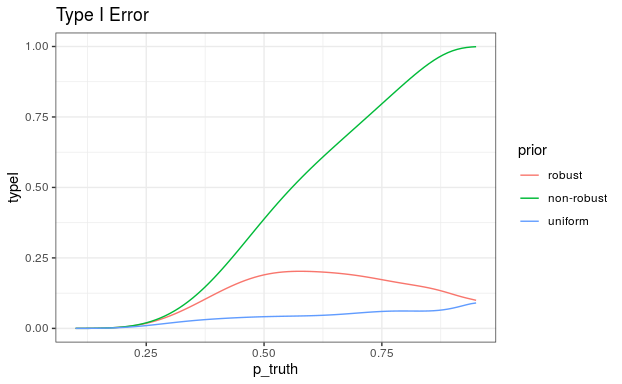### Power

The power demonstrates the gain of using an informative prior; i.e. 80% power is reached for smaller $$\delta$$ values in comparison to a design with non-informative priors for both arms.

delta <- seq(0,0.7,by=0.01)
m <- summary(map)["mean"]
p_truth1 <- m +   delta
p_truth2 <- m + 0*delta

power_uniform   <- design_uniform(  p_truth1, p_truth2)
power_nonrobust <- design_nonrobust(p_truth1, p_truth2)
power_robust    <- design_robust(   p_truth1, p_truth2)

ocP <- rbind(data.frame(p_truth1=p_truth1, p_truth2=p_truth2, delta=delta, power=power_robust,    prior="robust"),
data.frame(p_truth1=p_truth1, p_truth2=p_truth2, delta=delta, power=power_nonrobust, prior="non-robust"),
data.frame(p_truth1=p_truth1, p_truth2=p_truth2, delta=delta, power=power_uniform,   prior="uniform")
)

qplot(delta, power, data=ocP, colour=prior, geom="line", main="Power")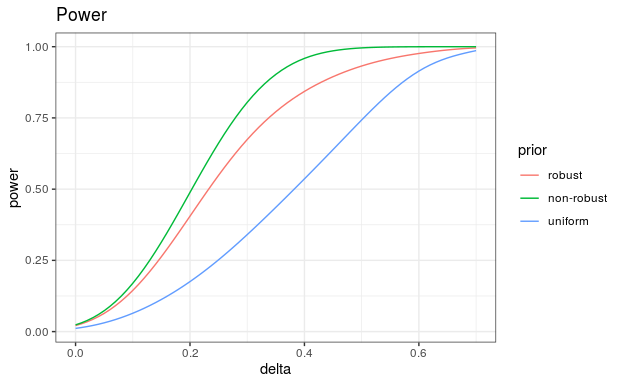### Data Scenarios

An alternative approach to visualize the study design to non-statisticians is by considering data scenarios. They show the decisions based on potential trial outcomes. The information needed are the critical values at which the decision criterion flips. In the 2-sample case this means to calculate the decision boundary, see the decision2S_boundary help for more information.

## Critical values at which the decision flips are given conditional
## on the outcome of the second read-out; as we like to have this as a
## function of the treatment group outcome, we flip label 1 and 2
decision_flipped <- decision2S(0.95, 0, lower.tail=TRUE)
crit_uniform     <- decision2S_boundary(uniform_prior, uniform_prior, 6, 24, decision_flipped)
crit_nonrobust   <- decision2S_boundary(map          , treat_prior  , 6, 24, decision_flipped)
crit_robust      <- decision2S_boundary(map_robust   , treat_prior  , 6, 24, decision_flipped)
treat_y2 <- 0:24
## Note that -1 is returned to indicated that the decision is never 1
ocC <- rbind(data.frame(y2=treat_y2, y1_crit=crit_robust(treat_y2),    prior="robust"),
data.frame(y2=treat_y2, y1_crit=crit_nonrobust(treat_y2), prior="non-robust"),
data.frame(y2=treat_y2, y1_crit=crit_uniform(treat_y2),   prior="uniform")
)

qplot(y2, y1_crit, data=ocC, colour=prior, geom="step", main="Critical values y1(y2)")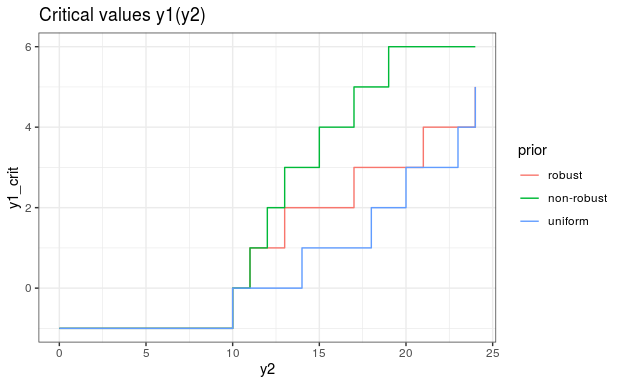The graph shows that the decision will always be negative if there are less than 10 events in the treatment group. On the other hand, under a non-robust prior and assuming 15 events in the treatment group, three (or less) placebo events would be needed for success. To check this result, we can directly evaluate the decision function:

## just positive
decision(postmix(treat_prior, n=24, r=15), postmix(map, n=6, r=3))
##  1
## negative
decision(postmix(treat_prior, n=24, r=14), postmix(map, n=6, r=4))
##  0

# Trial Analysis

Once the trial has completed and data is collected, the final analysis can be run with RBesT using the postmix function. Calculations are performed analytically as we are in the conjugate mixture setting.

r_placebo <- 1
r_treat   <- 14

## first obtain posterior distributions...
post_placebo <- postmix(map_robust,  r=r_placebo, n=6)
post_treat   <- postmix(treat_prior, r=r_treat  , n=24)

## ...then calculate probability that the difference is smaller than
## zero
prob_smaller <- pmixdiff(post_treat, post_placebo,  0, lower.tail=FALSE)

prob_smaller
##  0.9913125
prob_smaller > 0.95
##  TRUE
## alternativley we can use the decision object
decision(post_treat, post_placebo)
##  1

### R Session Info

sessionInfo()
## R version 3.6.1 (2019-07-05)
## Platform: x86_64-pc-linux-gnu (64-bit)
## Running under: Ubuntu 16.04.6 LTS
##
## Matrix products: default
## BLAS:   /usr/lib/libblas/libblas.so.3.6.0
## LAPACK: /usr/lib/lapack/liblapack.so.3.6.0
##
## locale:
##  C
##
## attached base packages:
##  stats     graphics  grDevices utils     datasets  methods   base
##
## other attached packages:
##  tidyr_1.0.0     scales_1.0.0    ggplot2_3.2.1   purrr_0.3.3
##  dplyr_0.8.3     bayesplot_1.7.0 knitr_1.25      RBesT_1.6-1
##  Rcpp_1.0.2
##
## loaded via a namespace (and not attached):
##   rstan_2.19.2       tidyselect_0.2.5   xfun_0.10
##   reshape2_1.4.3     vctrs_0.2.0        colorspace_1.4-1
##   htmltools_0.4.0    stats4_3.6.1       loo_2.1.0
##  yaml_2.2.0         rlang_0.4.0        pkgbuild_1.0.6
##  pillar_1.4.2       glue_1.3.1         withr_2.1.2
##  lifecycle_0.1.0    matrixStats_0.55.0 plyr_1.8.4
##  stringr_1.4.0      munsell_0.5.0      gtable_0.3.0
##  mvtnorm_1.0-11     codetools_0.2-16   evaluate_0.14
##  labeling_0.3       inline_0.3.15      callr_3.3.2
##  ps_1.3.0           parallel_3.6.1     highr_0.8
##  rmarkdown_1.16     R6_2.4.0           compiler_3.6.1